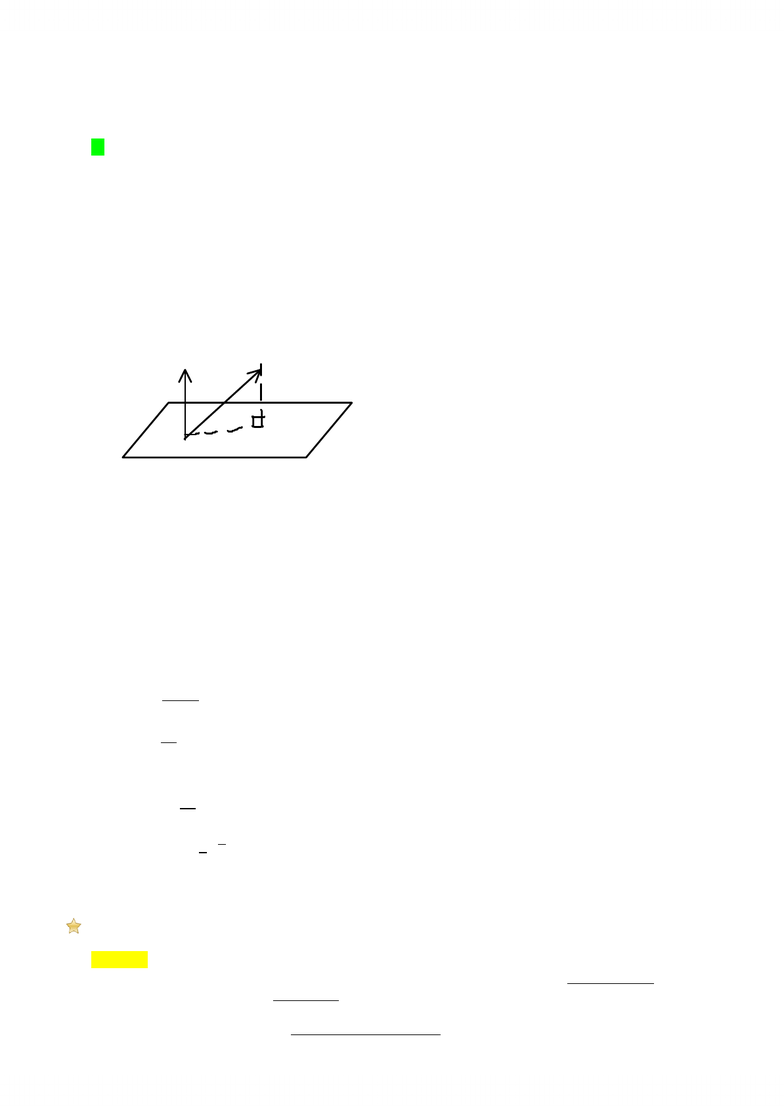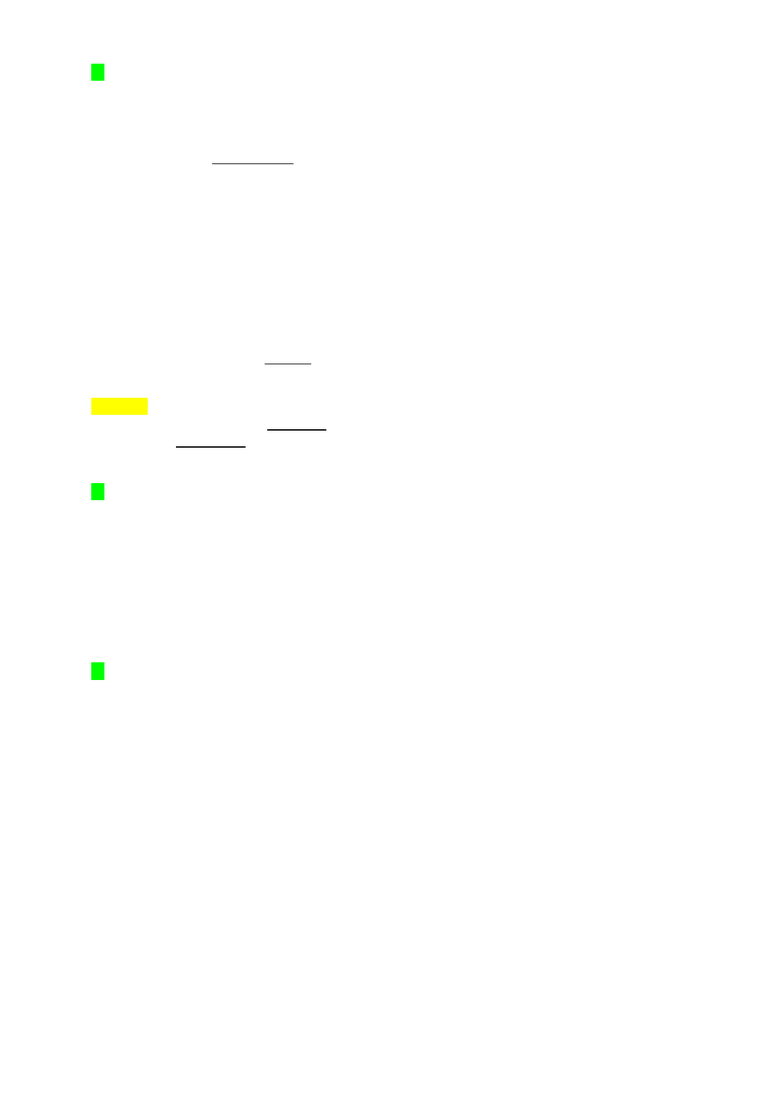Get 2 days of unlimited access
Class Notes (1,000,000)
CA (600,000)
UW (20,000)
MATH (2,000)
MATH136 (300)
Lecture 7

# Week 3 (lecture 7-9) Lecture Notes

Department
Mathematics
Course Code
MATH136
Professor
Patrick Roh
Lecture
7

This preview shows pages 1-3. to view the full 10 pages of the document.Eg
Find the shortest distance from the point to the plane 
Note:
This plane does NOT pass through the origin.
The vector

does NOT go from the plane to .
The normal vector of the plane is

We need a vector from ANY point on the plane to .
The point is on the plane











Systems of linear equations
Definition
An equation of the form for constants is called a linear equation.
The constants are the coefficients of the variables .
A set of linear equations is called a system of linear equations.
(Note: A linear equation can be represented geometrically as a hyperplane.)
n
Q


Lec 7 - Shortest paths and System of Linear Equations
Wednesday, January 18, 2012
9:33 AM
MATH 136 Page 1

Only pages 1-3 are available for preview. Some parts have been intentionally blurred.Eg
is a linear equation.
is NOT a linear equation.
is a linear equation. (should be written as .)
(This is known as the standard form)
In general, we will be looking at systems of linear equations in variables:



Where is the coefficient of variable in the equation .
Any vector
is a solution of the system if the equations are satisfied when
Definition
A system of linear equations is consistent if there is at least one solution.
Otherwise it is inconsistent.
Eg
This system has
as a solution.
The system is consistent.
Geometrically, we have two planes in that intersect. The point is one of the points of intersection.
Eg


No solutions. System is inconsistent.
Geometrically, we have two lines in that are parallel and thus do NOT intersect.
In general, a solution of a system of linear equations in variables is a point in where hyperplanes represented
by the linear equations, intersect.
We could have a system with
No solution. (Inconsistent)
1.
Eg parallel hyper planes.
One solution.
2.
All the hyperplanes intersect at a single point.
Eg Intersection of the planes is a line.
More than one solution
3.
Can you create a system with exactly 2 solutions?
MATH 136 Page 2

Only pages 1-3 are available for preview. Some parts have been intentionally blurred.Can you create a system with exactly 2 solutions?
Theorem: If the following system of linear equations:



has two distinct solutions
and
Then

is a solution for any and each gives a different solution.
(A consistent system with more than one solution has infinitely many solutions.)
Proof:
For each equation :










is a solution to the system for any
.
Assume , but
Thus


Since
then , a contraction.
Thus each gives a different solution.
MATH 136 Page 3
###### You're Reading a Preview

Unlock to view full version